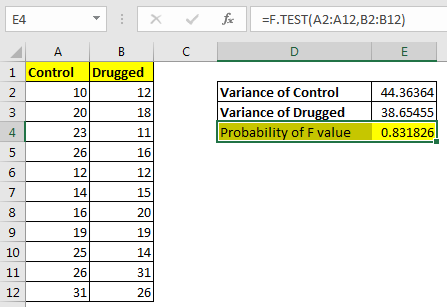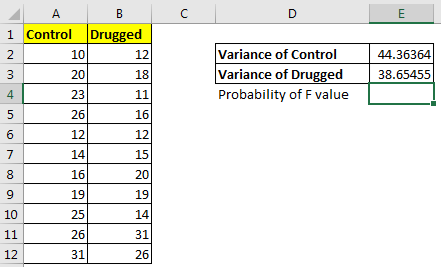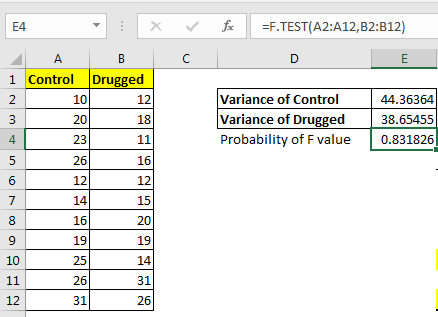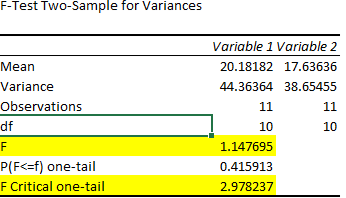# How to use Excel F.TEST Function in Excel

The F.TEST Function is used to calculate F statistic of two samples in excel internally and returns the two tailed probability of the of the F statistic under Null Hypothesis (H0). Note that F.TEST function does not returns the F test value, instead it returns it’s probability. If F.TEST returns value less then 0.05, we reject the null hypothesis.(Null Hypothesis (H0): the variance of two samples are not significantly different)
Syntax

 =F.TEST(array1, array2)

Array1: first sample of values. Mostly control sample.
Array2: second sample of values.
Let’s see an example to make things clear.

## Example: Check if there is significant difference in variances of drugged students and control students

Here I have some data, where some students were given a drug and some don’t. I compared there data and evaluated the variance in each group.We can see the difference in variance. But we want to check the probability of it. If it is less than 0.05 we will say there is a difference in variances else we will say there is no significant difference in variances of two groups.
Using F.TEST function, we calculate the two tailed probability.

 =F.TEST(A2:A12,B2:B12)

It returns 0.83, which is much greater than 0.05. Hence we can’t reject the null hypothesis.How it works?
Let’s see how would we have got  F Test of data analysis.And this how we would have interpreted it.

Well, if we calculate the F statistic (greater variance / smaller variance) we get 1.147. The critical value is 2.978. (Calculated using data analysis tool in excel). We can see that F value is less then F critical value, hence we can’t reject the null hypothesis.

This same thing we did just using one function F.TEST in excel. If the value is greater than 0.05 then we don’t reject the null hypothesis else we reject it.

So yeah guys, this how we use F.TEST function in excel. The F.TEST function was introduced in Excel 2010. In earlier versions it was available as FTEST. Although the FTEST function is still available in excel, excel recommends to use F.TEST function.

Terms and Conditions of use

The applications/code on this site are distributed as is and without warranties or liability. In no event shall the owner of the copyrights, or the authors of the applications/code be liable for any loss of profit, any problems or any damage resulting from the use or evaluation of the applications/code.